July 17, 2019

# Maximum Flow

• Chapter 26 of Introduction to Algorithms (3rd Edition), Thomas H. Cormen, Charles E. Leiserson, Ronald L. Rivest, and Clifford Stein.

# Maximum Flow

• We can use a directed graph to model a flow network
• A material is produced. It goes from a source to a sink
• The source produces the material to a fix rate
• At the sink, the material is consumed at the same rate
• The flow of the material at any point of the system is the rate at which that material moves

# Maximum Flow

• When can we use flow networks
• Flow networks can be used to model
• Liquids moving through tubes
• Parts of ensemble lines
• Electricity through power lines
• Information through communication networks, etc.

# Maximum Flow

• Directed edges in the flow network lead the material
• Each duct has a capacity
• The maximum rate to which the material flows
• i.e. 200 galons of liquid per hour in a tube
• i.e. 20 amps of electric current in a cable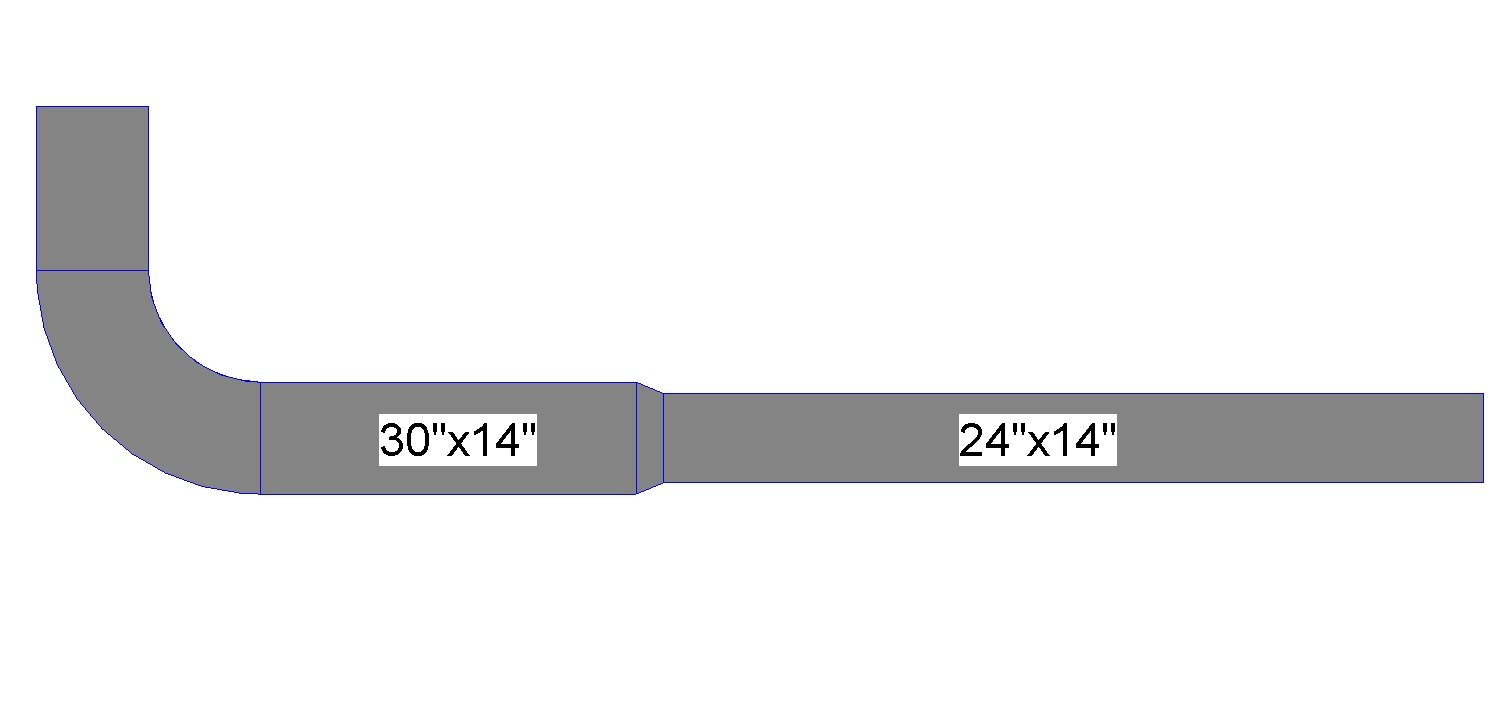# Maximum Flow

• Vertices are the joints in the duct, different from the source and sink
• Vertices do not collect material# Maximum Flow

• The material input rate is equal to the material output rate
• Flow conservation property
• Equivalent to the Kirchhoff current law, when the material is electric current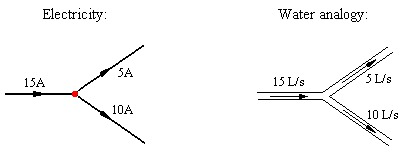# Maximum Flow

• Compute the highest rate to which the material can be moved
• From a source to a sink
• Without breaking any capacity restriction
• One of the simplest problems related to flow networks
• Can be solved with efficient algorithms

# Modeling the Problem

• Graph-based definition for flow networks
• Flow network
• $$G = (V, E)$$ is a directed graph in which each edge $$(u, v) \in E$$ has a non-negative capacity $$c(u, v) \geq 0$$.
• If $$(u, v) \notin E$$, we assume that $$c(u, v) = 0$$.
• We distinghish two types of vertices
• a source s
• a sink t
• We assume that each vertex is in some path from the source to the sink
• $$\forall v \in V$$, there exists a path $$s \rightarrow v \rightarrow t$$
• The graph is connected and $$|E| \geq |V| - 1$$

# Flow Network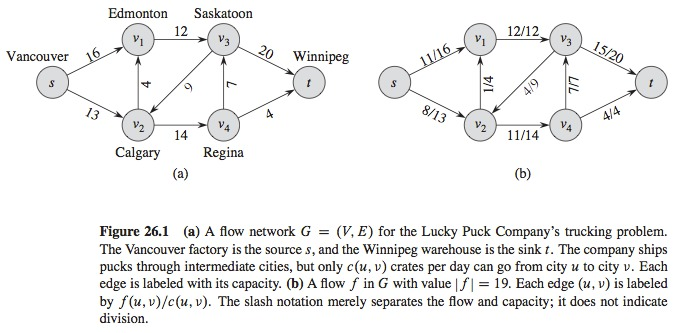# Flow

• Given $$G = (V, E)$$ we can represent a flow network with capacity function $$c$$
• Let $$s$$ be the source of the network and let $$t$$ be the sink
• A flow in $$G$$ is a function with real values $$f: V x V \rightarrow R$$ that satisfies the following three properties
• 1- Capacity restriction: $$\forall u, v \in V$$, we require $$0 \leq f(u, v) \leq c(u, v)$$
• 2- Bias simmetry: $$\forall u, v \in V$$, we require $$f(u, v) = -f(v, u)$$

# Flow

• The third property…
• 3- Flow conservation: $$\forall u \in V - \{s, t\}$$, we require:

• $$\sum\limits_{v \in V} f(v, u) = \sum\limits_{v \in V} f(u, v)$$
• The quantity $$f(u, v)$$, which can be positive, cero, or negative, is called the flow from vertex $$u$$ to vertex $$v$$
• If $$(u, v) \notin E$$, there can’t be flow from $$u$$ to $$v$$, then $$f(u, v) = 0$$.

# Flow

• The value of a flow is defined as:
• $$|f| = \sum\limits_{v \in V} f(s, v)$$
• The total flow that comes from the source
• $$| |$$ denotes the value of flow, NOT absolute value, nor cardinality
• The Problem of Maximum Flow
• We are given a flow network $$G$$ with source $$s$$ and sink $$t$$ and we want to find a maximum flow value

# Capacity Restriction

• The flow from a vertex to another should not exceed the given capacity

# Bias Simmetry

• The notation says that:
• The flow from a vertex $$u$$ to a vertex $$v$$ is the non negative of the flow in opposite direction

# Flow Conservation

• The total flow comming from a vertex, different from the source or sink, is 0

# Example of Flow# Networks with Multiple Sources and Sinks

• There can be several sources and sinks
• $${s_1, s_2, \dots, s_m}$$
• $${t_1, t_2, \dots, t_n}$$
• This problem is not more difficult than the previous one
• Can be reduced to an ordinal maximum flow problem
• Super-source
• Super-sink

# Networks with Multiple Sources and Sinks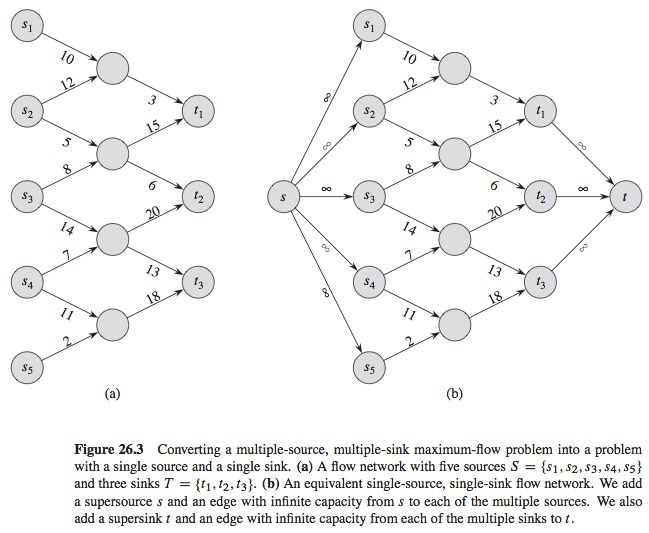# Working with Flows

• Summation notation is implicit
• $$\sum\limits_{x \in X}\sum\limits_{y \in Y} f(x, y)$$
• Conservation flow can be expressed as
• $$f(u, V) = 0$$ for all $$u \in V - \{s, t\}$$
• In $$f(s, V - s) = f(s, V)$$, the term $$V - s$$ refers to the set $$V - \{s\}$$

# The Ford-Fulkerson Method

• Three important ideas, relevant for many flow algorithms and problems
• Residual networks
• Augmenting paths
• Cuts
• Different implementations of the algorithm with different execution times

# The Ford-Fulkerson Method

• Iterative method
• Starts with $$f(u, v) = 0$$ for all $$u, v \in V$$, with an initial flow value of 0
• At each iteration, we increment the flow value when we find an “augmenting path”: a path from the source $$s$$ to the sink $$t$$ that we can use to send flow
• We repeat this process until we cannot find any augmenting path
• The theorem of maximum-flow minimum-cut shows that, at the end, the process takes a maximum flow

# The Ford-Fulkerson Method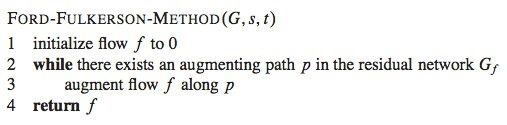# Residual Networks

• Intuitivelly
• Given a flow network and a flow, the residual network consists of edges that can admit more flow

# Residual Networks

• Formally
• Given a flow network $$G = (V, E)$$ with source $$s$$ and sink $$t$$
• Let $$f$$ be a flow in $$G$$, and considering a pair of vertices $$u, v \in V$$
• The amount of additional flow that we can take from $$u$$ to $$v$$ without exceding capacity $$c(u, v)$$ is the residual capacity of $$(u, v)$$ given by $$c_f(u, v) = c(u, v) - f(u, v)$$
• Given a flow network $$G = (V, E)$$ and a flow $$f$$, the residual network of $$G$$ induced by $$f$$ is $$G_f = (V, E_f)$$, where
• $$E_f = \{(u, v) \in V x V : c_f(u, v) > 0\}$$

# Example of Residual Network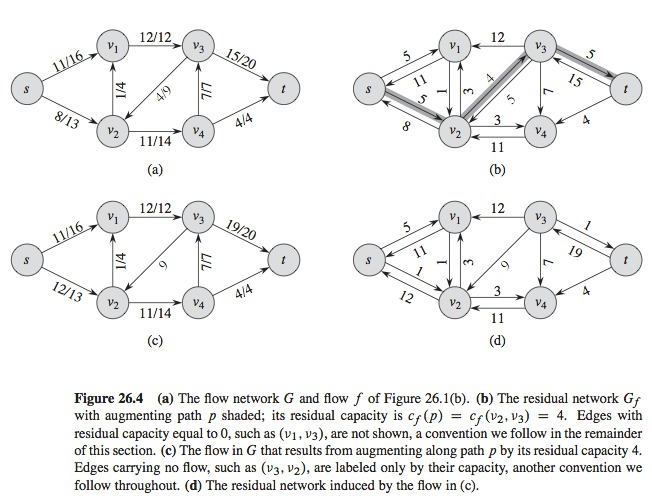# Augmenting Paths

• Given a flow network $$G = (V, E)$$ and a flow $$f$$
• An augmenting path $$p$$ is a path from $$s$$ to $$t$$ in the residual network $$G_f$$
• Figure 26.4b
• We can increase the flow in 4 units, $$c_f(v_2, v_3) = 4$$
• The residual capacity of the path is 4 without violating the capacity restriction, the lowest residual capacity is 4: $$c_f(p) = min\{c_f(u, v) : (u, v) \in p\}$$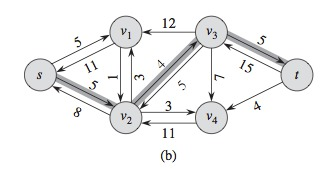# Lemma 26.3: Augmenting Paths

• Given $$G = (V, E)$$, a flow network
• Let $$f$$ be a flow in $$G$$
• Given $$p$$ an augmenting path in $$G_f$$
• Define a flow $$f_p$$ in $$G_f$$ as the function $$f_p : V x V \rightarrow R$$ by:

$$f_p(u, v) = \begin{cases} c_f(p) &\mbox{if } (u, v) \in p\\ -c_f(p) &\mbox {if } (v, u) \in p\\ 0 &\mbox{any other way}\\ \end{cases}$$

• Then $$f_p$$ is a flow in $$G_f$$ with value $$|f_p| = c_f(p) > 0$$

# Corolary 26.4

• Corolary 26.4 tells us that, if we add $$f_p$$ to $$f$$, we obtain another flow in $$G$$ with a value closer to the maximum
• Let $$G = (V, E)$$ be a flow network
• Let $$f$$ be a flow in $$G$$
• Let $$p$$ be an augmenting path in $$G_f$$
• Let $$f_p$$ be defined as previous (previous page)
• We define a function $$f': V x V \rightarrow R$$ by $$f'=f + f_p$$
• Then, $$f'$$ is a flow in $$G$$ with value $$|f'| = |f| + |f_p| > |f|$$

# Cuts in Flow Networks

• The flow is maximum iff its residual network does not contain an augmenting path
• To show this we need the cut notion
• A cut $$(S, T)$$ of a flow network $$G = (V, E)$$ is a partition of $$V$$ in $$S$$ and $$T = V - S$$ such that $$s \in S$$ and $$t \in T$$
• The Net Flow through the $$cut(S, T)$$ is defined as $$f(S, T)$$
• The Capacity of $$cut(S, T)$$ is $$c(S, T)$$
• A minimum cut of a network is a cut with minimum capacity over all cuts in the network

# Example of Cut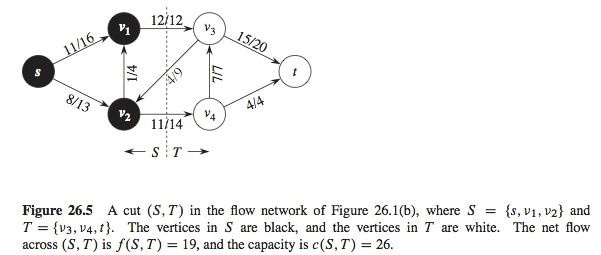• Net flow
$$f(v_1,V_3) + f(v_2,v_4) - f(v_3,v_2) = 12 + 11 - 4$$
$$= 19$$
• Capacity
$$c(v_1,v_3) + c(v_2,v_4) = 12 + 14$$
$$=26$$

# Lemma 26.5

• The net flow through any cut is the same and equal to the value of the flow
• Given $$f$$, the flow in a flow network $$G$$ with source $$s$$ and sink $$t$$, and let $$(S, T)$$ be a cut of $$G$$
• Then the net flow through $$(S, T)$$ is $$f(S, T) = |f|$$

# Corolary 26.6

• The value of any flow $$f$$ in a flow network $$G$$ is bounded from above by the capacity of any cut of $$G$$

# Theorem of the Maximum-Flow Minimum-Cut

• The value of a maximum flow is equal to the capacity of a minimum cut
• If $$f$$ is a flow in a network flow $$G = (V, E)$$ with source $$s$$ and sink $$t$$, then the following conditions are equivalent:
• $$f$$ is a maximum flow in $$G$$
• The residual network $$G_f$$ does not contain augmenting paths
• $$|f| = c(S, T)$$ for any cut $$(S, T)$$ of $$G$$

# Basic Ford-Fulkerson Algorithm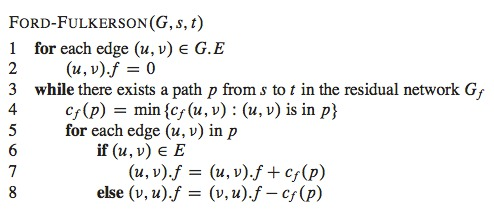# Example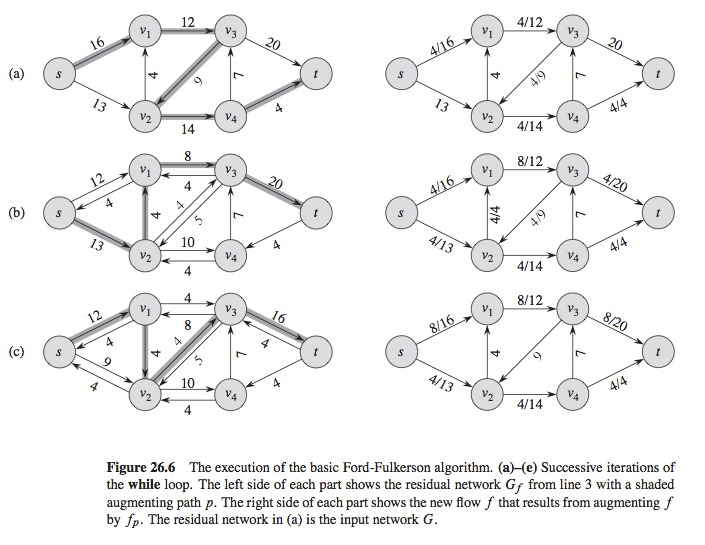# Example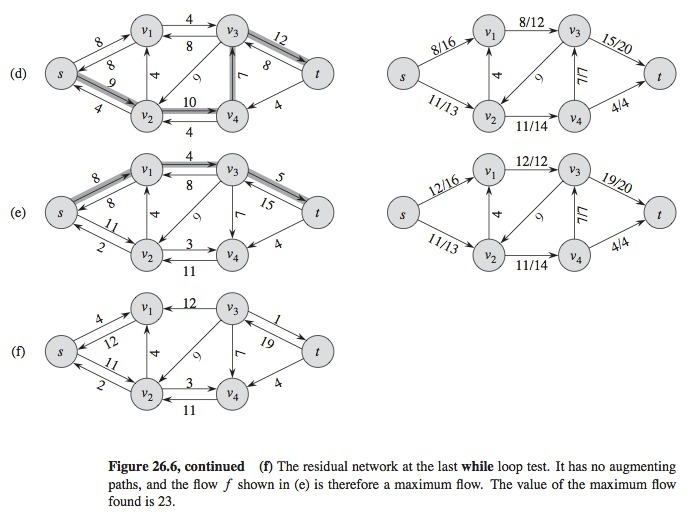# Analysis of Ford-Fulkerson

• Depends on a method to find the augmenting path
• The augmenting path is the shortest one from $$s$$ to $$t$$
• The total number of augmentings is $$O(VE)$$, $$O(E)$$ per augmenting. Then, the algorithm has an execution time of $$O(VE^2)$$
• Generic-Push-Relabel takes $$O(V^2E)$$
• Relabel-to-Front takes $$O(V^3)$$